hasChanged

Detect change in data since last time step

Description

example

tf = hasChanged(data_name) returns 1 (true) if the value of data_name at the beginning of the current time step is different from the value of data_name at the beginning of the previous time step. Otherwise, the operator returns 0 (false).

The argument data_name can be:

• A scalar variable.

• A matrix or an element of a matrix.

• If data_name is a matrix, the operator returns true when it detects a change in any element of data_name.

• Index elements of a matrix by using numbers or expressions that evaluate to a constant integer. See Supported Operations for Vectors and Matrices.

• A structure or a field in a structure.

• Any valid combination of structure fields or matrix elements.

The argument data_name cannot be a nontrivial expression or a custom code variable.

Note

Standalone charts in MATLAB® do not support change detection on an element of a matrix or a field in a structure.

Examples

expand all

Transition out of state if any element of the matrix M has changed value since the last time step or input event.

[hasChanged(M)]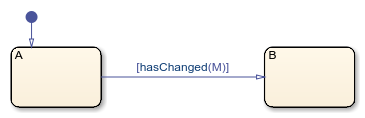Transition out of state if the element in row 1 and column 3 of the matrix M has changed value since the last time step or input event.

In charts that use MATLAB as the action language, use:

[hasChanged(M(1,3))]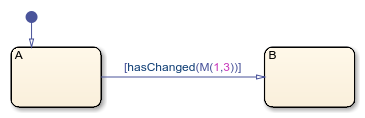In charts that use C as the action language, use:

[hasChanged(M)]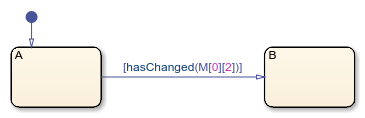Transition out of state if any field of the structure struct has changed value since the last time step or input event.

[hasChanged(struct)]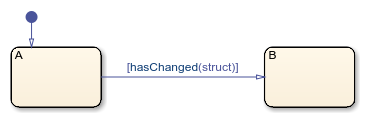Transition out of state if the field struct.field has changed value since the last time step or input event.

[hasChanged(struct.field)]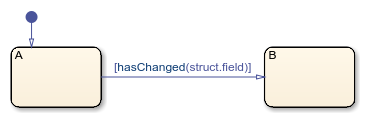Tips

• If multiple input events occur in the same time step, the hasChanged operator can detect changes in data value between input events.

• If the chart writes to the data object but does not change the data value, the hasChanged operator returns false.

• The type of Stateflow® chart determines the scope of the data supported by the change detection operators:

• Standalone Stateflow charts in MATLAB: Local only

• In Simulink® models, charts that use MATLAB as the action language: Input only

• In Simulink models, charts that use C as the action language: Input, Output, Local, or Data Store Memory

• In a standalone chart in MATLAB, a change detection operator can detect changes in data specified in a call to the step function because these changes occur before the start of the current time step. For example, if x is equal to zero, the expression hasChanged(x) returns true when you execute the chart ch with the command:

step(ch,'x',1);
In contrast, a change detection operator cannot detect changes in data caused by assignments in state or transition actions in the same time step. Instead, the operator detects the change in value at the start of the next time step.

• In a chart in a Simulink model, if you enable the chart option Initialize Outputs Every Time Chart Wakes Up, using an output as the argument of the hasChanged operator always returns false. For more information, see Initialize outputs every time chart wakes up.

• When row-major array layout is enabled in charts that use hasChanged, code generation produces an error. Before generating code in charts that use hasChanged, enable column-major array layout. See Select Array Layout for Matrices in Generated Code.

Introduced in R2007a

Stateflow DocumentationGet trial now